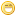The beta method programJmsNxnUltimate FellowPosts: 1,214 Threads: 126 Joined: Dec 2010 02/25/2022, 03:05 AM (This post was last modified: 02/25/2022, 03:13 AM by JmsNxn.) Hey, everyone! I thought I'd post a link to my github repository for the beta.gp program. I will post the readme of the program and the file itself, along with the github. If only I feel the need to update or fix some errors in my code; I'll do it on github, and not necessarily on this post. Here is the readme.txt which gives a run down of everything: Code:`# The-Beta-Method-Thesis The final program for the beta method of iterated exponentials Coded by James David Nixon, with supplementary code by Sheldon Levenstein and Mike3 of the tetration forum. Levenstein optimized some protocols I had written, and we use Mike3's graphing protocol. To begin use of the program one must execute the file in a pari-gp shell. We can now choose digit precision by taking "\p d" and series precision by "\ps count"; for d,count positive integers. From here, one must call init(l,b); or init_OFF(l,b) if abs(b) > 1E6, abs(b)<1E-4. The variable l must have a real part greater than zero; and b cannot equal zero (this produces the trivial beta), and the trivial tetration Sexp = 1. After initialization, the values l = mult and b = base are global variables. It is possible to initialize these objects as polynomials as well; meaning mult = 1+u or base = 1+v are perfectly valid. This protocol internally uses the variable x, so do not use this variable or reassign this variable else where in the program. This produces 4 function protocols (and a few variations): beta(z) = exp(base * beta(z-1))/(1+exp(-mult * (z-1))) tau(z) = log(1+tau(z+1)/beta(z+1))/base - log(1+exp(-mult * z))/base Sexp_N(z+1) = beta(z+1) + tau(z+1) = exp(base * (beta(z) + tau(z))) Each of these functions has a period of 2 * Pi * I/mult. They will be accurate to the digit precision, usually; if not, re run the initialization protocol with a higher series precision. Or one can keep the series precision and run init(l,b,COUNT) (resp. init_OFF); where COUNT determines how many iterations to do. One should never have to exceed 1000. There is an optional parameter in tau and Sexp_N which can tell us how many times to do the iteration; the program by default chooses enough for most calculations; sometimes it may be beneficial to induce more iterations. We can treat these three functions as polynomials as well; so that beta(1+z), tau(1+z), Sexp_N(1+z), are all viable prompts, which produce the polynomial about 1 in z to the desired series precision. There is also a separate initialization protocol you can run after the first which gives the summative form of the error. This would be: init_rho(c, {LIMIT = default(seriesprecision)}) which creates up to the series precision a sequence of functions rho, which act as sum(j=1,LIMIT,RHO[j]) = tau(q+CENTER,LIMIT-2). The variable CENTER=c; and this is declared as a global constant. This protocol internally uses the variable q; so it is best warned to not use the variable q elsewhere in the program. This spawns the function: rho(z,j) which is an evaluation of a polynomial centered about CENTER upto the series precision. The variable j indexes up until how many rho terms were made using LIMIT in init_rho. Or, if there's an overflow it sets the protocol to zero; or if j is outside of the index list, sets the protocol to zero. This satisfies: rho(z,0) = 0; rho(z,1) = -log(1+exp(-mult * z))/base sum(j=0,LIMIT-1, rho(z,j)) = tau(z, LIMIT-2) rho(z,j) = tau(z,j-1) - tau(z,j-2); And lastly, a protocol: tau_rho(z) = tau(z,LIMIT-2); Which is just short hand for the sum. /*****************************************************************************************************/ The next functions are a Julia set protocol for beta. We want to determine the points that are in the weak Julia set, and the points which are not. These are points where the orbits escape. A point a belongs to the weak Julia set if, for any D> 0: limsup n to infinity sup(|z-a|

 Possibly Related Threads… Thread Author Replies Views Last Post Code to calculate tetration using my method Shanghai46 10 899 10/19/2023, 09:15 PM Last Post: marracconew fatou.gp program sheldonison 33 65,673 07/10/2022, 02:23 AM Last Post: Catullus My new ABEL_L.gp program JmsNxn 13 10,336 10/06/2021, 07:18 PM Last Post: Ember Edison Natural complex tetration program + video MorgothV8 1 6,592 04/27/2018, 07:54 PM Last Post: MorgothV8 Mathematica program for tetration based on the series with q-binomial coefficients Vladimir Reshetnikov 0 5,288 01/13/2017, 10:51 PM Last Post: Vladimir Reshetnikov complex base tetration program sheldonison 23 85,186 10/26/2016, 10:02 AM Last Post: Gottfried C++ program for generatin complex map in EPS format MorgothV8 0 5,579 09/17/2014, 04:14 PM Last Post: MorgothV8 Which method is currently "the best"? MorgothV8 2 9,661 11/15/2013, 03:42 PM Last Post: MorgothV8 "Kneser"/Riemann mapping method code for *complex* bases mike3 2 12,368 08/15/2011, 03:14 PM Last Post: Gottfried An incremental method to compute (Abel) matrix inverses bo198214 3 15,631 07/20/2010, 12:13 PM Last Post: Gottfried

Users browsing this thread: 1 Guest(s)